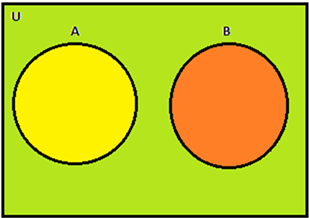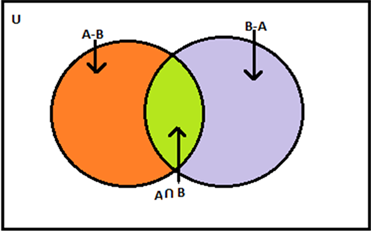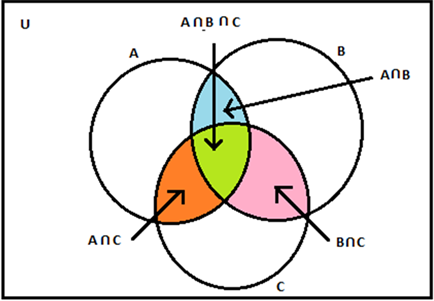# Union And Intersection Of Sets

Cardinal Number of a set

The number of distinct elements or members in a finite set is known as the cardinal number of a set. Basically, through cardinality, we define the size of a set. The cardinal number of a set A is denoted as n(A), where A is any set and n(A) is the number of members in set A.

Consider a set A consisting of the prime numbers less than 10.

Set A ={2, 3, 5, 7}.

As the set A consists of 4 elements, therefore, the cardinal number of set A is given as n(A) = 4.

## Properties related to difference, union and intersection and the cardinal number of set

i) Union of Disjoint Sets:

If A and B are two finite sets and if A ∩ B = ∅, then

n(A ∪ B) = n(A) + n(B)

In simple words if A and B are finite sets and these sets are disjoint then the cardinal number of Union of sets A and B is equal to the sum of the cardinal number of set A and set B.Figure 1- Disjoint sets

The union of the disjoint sets A and B represented by the Venn diagram is given by A ∪ B and it can be seen that A ∩ B = ∅ because no element is common to both the sets.

ii) Union of two sets:

If A and B are two finite sets, then

n(A ∪ B) = n(A) + n(B) – n(A ∩ B)

Simply, the number of elements in the union of set A and B is equal to the sum of cardinal numbers of the sets A and B, minus that of their intersection.Figure 2- Union of two sets

In the figure given above the differently shaded regions depict the different disjoint sets i.e. A – B, B – A and A ∩ B are three disjoint sets as shown and the sum of these represents A ∪ B. Hence,

n (A ∪ B) = n (A – B) + n(B – A) + n(A ∩ B)

iii) Union of three sets

If A, B and C are three finite sets, then;

n(A ∪ B ∪ C) = n(A) + n(B) + n(C) – n(A ∩ B) – n(B ∩ C) – n(A ∩ C) + n(A ∩ B ∩ C)

This is clearly visible from the Venn diagram that the union of the three sets will be the sum of the cardinal number of set A, set B, set C and the common elements of the three sets excluding the common elements of sets taken in pairs of two.Figure 3-Union of three sets

## Video Lesson

### Applied Concept – Cardinality of Sets### Solved Example

Let us see an example to make our point clear.

Example: There is a total of 200 students in class XI. 120 of them study mathematics, 50 students study commerce and 30students study both mathematics and commerce. Find the number of students who

i) Study mathematics but not commerce

ii) Study commerce but not mathematics

iii) Study mathematics or commerce

Solution: The total number of students represents the cardinal number of the universal set. Let A denote the set of students studying mathematics and set B represent the students studying commerce.

Therefore,

n (U) = 200

n(A) = 120

n(B) = 50

n(A ∩ B) = 30

The Venn diagram represents the number of students studying mathematics and commerce.
i) Here, we are required to find the difference of sets A and B.

n(A) = n(A – B) + n(A ∩ B)

n(A-B) = n(A) – n(A ∩ B)
⇒ n (A – B) = 120 – 30 = 90

The number of students who study mathematics but not commerce is 90.

ii) Similarly here, we are required to find the difference of sets B and A

n (B) = n (B – A) + n (A ∩ B)
⇒ n (B – A) = 50 – 30 = 20

The number of students who study commerce but not mathematics is 20.

iii) The number of students who study mathematics or commerce

n (A ∪ B) = n(A) + n(B) – n(A ∩ B)

⇒ n(A ∪ B) = 120 + 50 – 30 = 140

For better learning and to excel in all spheres of life join BYJU’S. Happy Learning!!’

Test your knowledge on Union And Intersection Of Sets Cardinal Number Practical Problems